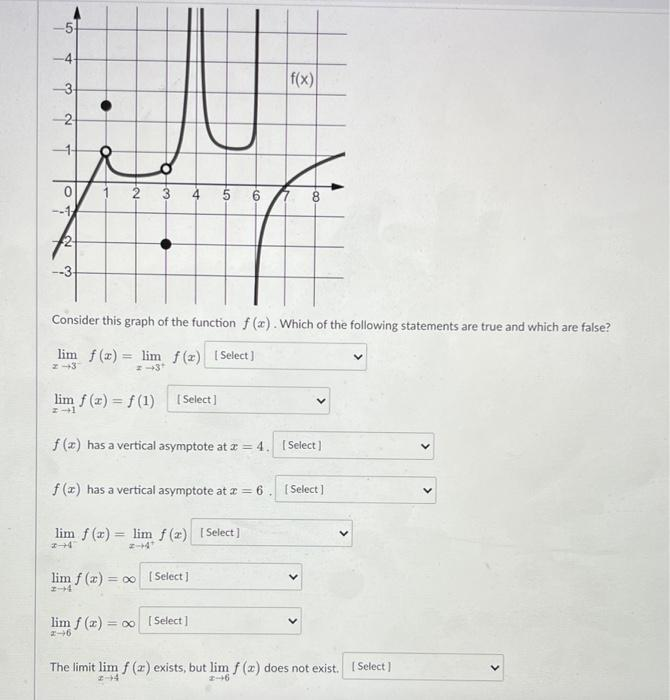# Question Solved1 Answer-5 -4 -3 f(x) 3 2 -1 0 --1 2 3 4 5 6 B --31 Consider this graph of the function f (). Which of the following statements are true and which are false? lim f(x) = lim f(x) [Select) 2-3 3 limf (a) = f (1) [Select] f () has a vertical asymptote at * = 4. Select) f (x) has a vertical asymptote at =6. Select ] lim f (x) = lim f (2) Select] - 4 -- lim f (x) = 0 [Select] lim f () = [Select] 16 The limit lim f (x) exists, but limf (2) does not exist. Select] 4 6Transcribed Image Text: -5 -4 -3 f(x) 3 2 -1 0 --1 2 3 4 5 6 B --31 Consider this graph of the function f (). Which of the following statements are true and which are false? lim f(x) = lim f(x) [Select) 2-3 3 limf (a) = f (1) [Select] f () has a vertical asymptote at * = 4. Select) f (x) has a vertical asymptote at =6. Select ] lim f (x) = lim f (2) Select] - 4 -- lim f (x) = 0 [Select] lim f () = [Select] 16 The limit lim f (x) exists, but limf (2) does not exist. Select] 4 6
More
Transcribed Image Text: -5 -4 -3 f(x) 3 2 -1 0 --1 2 3 4 5 6 B --31 Consider this graph of the function f (). Which of the following statements are true and which are false? lim f(x) = lim f(x) [Select) 2-3 3 limf (a) = f (1) [Select] f () has a vertical asymptote at * = 4. Select) f (x) has a vertical asymptote at =6. Select ] lim f (x) = lim f (2) Select] - 4 -- lim f (x) = 0 [Select] lim f () = [Select] 16 The limit lim f (x) exists, but limf (2) does not exist. Select] 4 6